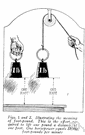# foot-pound

(redirected from Pound-foot)
Also found in: Thesaurus, Medical, Encyclopedia.

## foot-pound

(fo͝ot′pound′)
n. Abbr. ft-lb
A unit of work equal to the work done by a force of one pound acting through a distance of one foot in the direction of the force.

## foot-pound

n
(Units) an fps unit of work or energy equal to the work done when a force of 1 pound moves through a distance of 1 foot. Abbreviation: ft-lb

## foot′-pound′

n.
a unit of energy equal to the energy expended in raising one pound a distance of one foot. Abbr.: ft-lb
[1840–50]

## foot-pound

A unit of work equal to the work or energy needed to lift a one-pound weight a distance of one foot against the force of the Earth's gravity.
ThesaurusAntonymsRelated WordsSynonymsLegend:
 Noun 1foot-pound - a unit of work equal to a force of one pound moving through a distance of one footenergy unit, heat unit, work unit - a unit of measurement for workfoot-ton - 2240 foot-pounds
Site: Follow: Share:
Open / Close